Previous: 3.3.1 Energy Loss Mechanisms Up: 3.3 Monte-Carlo Method Next: 3.3.2.1 Interatomic Screening Potential

## 3.3.2 Nuclear Stopping Process

The interaction of a moving charged particle with nuclei of a solid can be described by a series of classical elastic scattering processes. It is assumed by the Monte-Carlo method that only two particles (the ion and one target nucleus) are considerably involved in one scattering processes. This is called the binary collision approximation), which reduces the multi particle problem to a classical elastic two particle problem which is characterized by the conservation of energy (3.60) and momentum (3.61) (3.62). The non-relativistic conservation laws can be used because the particle velocities are of the order of 0,1% of the speed of light for typical ion implantation applications.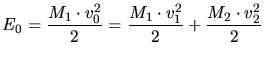(3.60)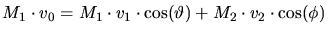(3.61)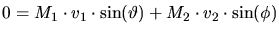(3.62)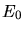is the initial kinetic energy of the two particle system,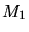,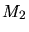are the masses of the particles.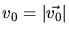is the speed of the moving particle before, while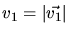is its velocity after the scattering process.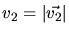is the final speed of the initially resting particle.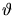and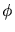are the deflection angles. Besides the initial energy and the initial momentum the scattering process is characterized by the impact parameterwhich is the minimum distance of the two particles if they would pass without interaction. Fig. 3.2 schematically shows the behavior of an elastic two particle scattering process.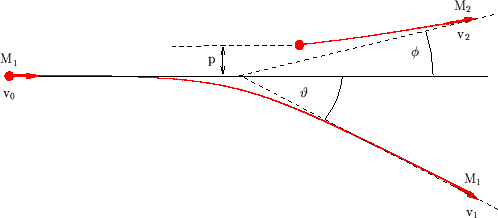By transforming the equations to the center-of-mass coordinates the two particle problem can be converted to that of a single particle moving in a central potential (Fig. 3.3).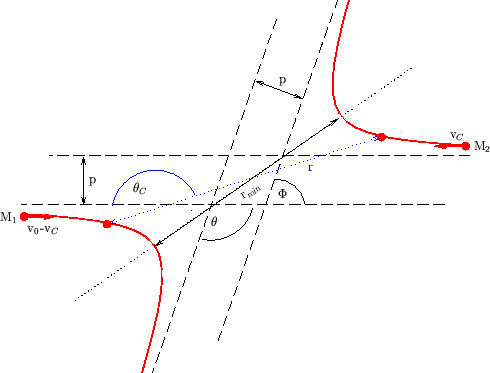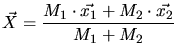(3.63)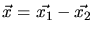(3.64)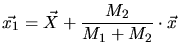(3.65)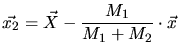(3.66)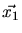,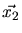are the coordinates in the laboratory system whileis the position of the center of mass and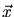are the relative coordinates of the particles. The velocity of the center-of-mass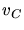is constant.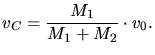(3.67)

Introducing a reduced mass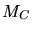(3.67) can be reformulated to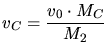(3.68)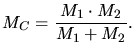(3.69)

The velocities of the particles in the center-of-mass system at the beginning of the scattering event are(particle 2) and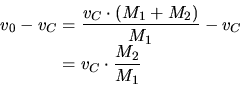(3.70)

(particle 1). The total momentum is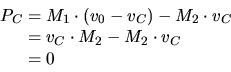(3.71)

and therefore the particle velocities remain unchanged after the collision. The kinetic energy of the particles is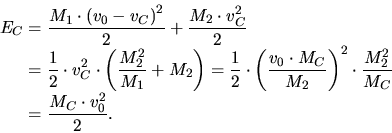(3.72)

Using these relations the kinetic energy transfer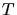from the incident particle to the target can be derived as a function of the scattering angle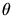(Fig. 3.3) which is related to the anglesandin the laboratory system (Fig. 3.2) by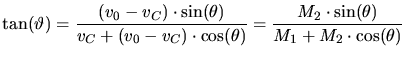(3.73)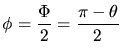(3.74)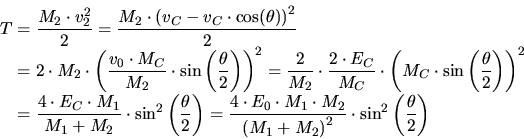(3.75)

In order to rigorously simulate the motion of a particle through mater not only the transfered energy as a function of the scattering angle but also the scattering angle as a function of the impact parameter has to be calculated, which requires a rigorous solution of the equation of motion. A very convenient way is the derivation by using Lagrangian mechanics. The Lagrange function in the center-of-mass coordinates ((3.63) and (3.64)) is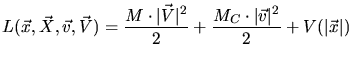(3.76)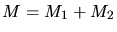(3.77)

Using the Lagrange equation (3.78) gives two independent equations of motion, one for the center-of-mass (3.79) and one for a particle in the central potential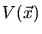(3.80), which represents the motion in the center-of-mass system.is equivalent to the interaction potential of the particles.(3.78)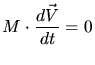(3.79)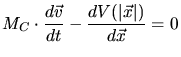(3.80)

For a particle moving in a central potential the energy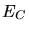and the angular momentum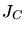is constant. Using these conservation law the equation of motion can easily be solved in polar coordinates. The initial energy and the initial angular momentum are(3.81)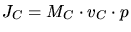(3.82)

while they can be described during the motion by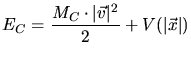(3.83)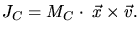(3.84)

(3.83) and (3.84) can be expressed in polar coordinates by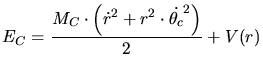(3.85)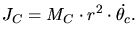(3.86)

Combining (3.83) and (3.84) with (3.81) and (3.82) gives the equation of motion in polar coordinates.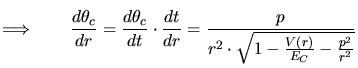(3.87)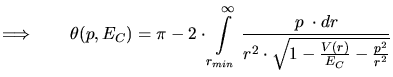(3.88)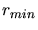is the distance of minimum approach between the particles and it is determined by the real root of the denominator of (3.87). An interpretation of the coordinates of the central potential scattering problem is denoted by the dotted arrow in Fig. 3.3.

The major problem that arises when modeling this scattering process is to determine the interaction potential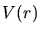between the ion and the target. In principle it is a repulsive Coulomb potential due to the positive charges of the cores. This Coulomb potential is screened by the surrounding electrons. The effect of the electrons can be described by adding a dimensionless screening function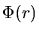to the Coulomb potential.(3.89)

SubsectionsPrevious: 3.3.1 Energy Loss Mechanisms Up: 3.3 Monte-Carlo Method Next: 3.3.2.1 Interatomic Screening Potential

A. Hoessiger: Simulation of Ion Implantation for ULSI Technology